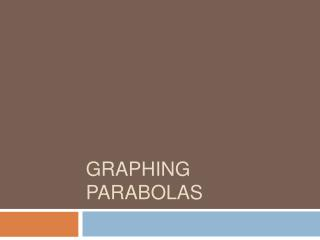DownloadDownload PresentationGraphing Parabolas

# Graphing Parabolas

Download Presentation## Graphing Parabolas

- - - - - - - - - - - - - - - - - - - - - - - - - - - E N D - - - - - - - - - - - - - - - - - - - - - - - - - - -
##### Presentation Transcript

1. Graphing Parabolas

2. Pattern Probes Which of the following relationships describes the pattern: 1,-1,-3,-5…? A. tn = -2n B. tn = -2n +3 C. tn = 2n – 3 D. tn = 2n

3. Pattern Probes 2. What is the 62th term of the pattern 1,9,17,25,…? A. 489 B. 496 C. 503 D. 207

4. Quadratics Patterns Note: Another phrase for linear pattern is an arithmetic sequence. • A linear pattern is given by tn = t1 +(n-1)d • A quadratic pattern has its CD on D2. • A linear pattern has a common difference on D1. • A quadratic pattern is defined by tn = an2 + bn +c • The CD = 2a for a quadratic Last time we learned that:

5. Let’s Graph (Put on your graphing shoes and graph in blue?) Quadratics, as we know, do not increase by the same amount for every increase in x. (How do we know this?) Therefore, their graphs are not straight lines, but curves. In fact, if you’ve ever thrown a ball, watched a frog jump, or taken Math 12, you’ve seen a quadratic graph! Its table of values would be Consider the function f(x) = x2 - the simplest quadratic (Why?) The pattern would be

6. Graphing Cont Domain: xε R Range: y ε [0,∞) or y ≥ 0 Axis of Symmetry: x = 0 Vertex Once we have the vertex, the rest of the points follow the pattern: Over 1, up 1 Over 2, up 4 Over 3, up 9 We can plot these points to get the PARABOLA

7. Transformations of y= x2 That parabola (which represents the simplest quadratic function), can be Reflected Stretched vertically Slid horizontally Slid vertically These are called transformations: Ref, VS, HT, VT, These show up (undone) in the transformational form of the equation: The form y = ax2 + bx + c is called the GENERAL FORM

8. Transformations Ex. Graph the function What are the transformations? So we can use these to get a MAPPING RULE: Reflection: No (the left side is not multiplied by -1) Vertical Stretch, VS? Yes VS = 2 Vertical Translational, VT? Yes VT = -3 Horizontal Translational, HT? Yes HT = 1 (x,y)  (x+1, 2y-3) We now take the old table of values and do what the mapping rule says.

9. Transformations Ex. Graph the function So we can use these to get a MAPPING RULE: (x,y)  (x+1, 2y-3) We now take the old table of values and do what the mapping rule says.

10. Transformations Ex. Graph the function New Vertex (1,-3) that is (HT, VT)

11. Transformations Ex. Graph the function Once we find the new vertex, the rest of the points follow the pattern Over 1, up 1 x VS Over 2, up 4 x VS Over 3, up 9 x VS New Vertex (1,-3) that is (HT, VT) Domain: x ε R Range: y ε [-3,∞) Axis of symmetry: x = 1

12. Graph the following. State the axis of symmetry, range, domain and the y-intercept of each. • A) • B) • C)

13. Domain: x ε R Range: y ε [3,∞) Axis of symmetry: x = 2 Y-int (0,11) A) Ref: No VS: 2 VT: 3 HT: 2 (x,y)(x+2,2y+3)

14. Domain: x ε R Range: y ε (-∞,0] Axis of symmetry: x = -1 Y-int (0,-0.5) B) Ref: Yes VS: 1/2 VT: 0 HT: -1 (x,y)(x-1,-0.5y)

15. Domain: x ε R Range: y ε (∞,4] Axis of symmetry: x = -6 Y –int (0,-32) C) Ref: Yes VS: 1 VT: 4 HT: -6 (x,y)(x-6,-y+4)

16. Going backwards Find the equation of this graph: Ans: 2(y+1)=(x-1)2

17. Going Backwards • What quadratic function has a range of y ε(-∞,6], a VS of 4 and an axis of symmetry of x = -5? • What quadratic function has a vertex of (2,-12) and passes through the point (1,-9)? a) b)

18. Solving for x using transformational form We can use transformational form to solve for x- values given certain y-values. Example: The parabola passes through the point (x,2). What are the values of x?

19. Solving for x using transformational form Find the x-intercepts of the following quadratic functions:

20. Changing Forms General Form: y = ax2 +bx + c Transformational Form: Standard Form:

21. What are you good for? Transformational Form: General Form: f(x)=y = ax2 +bx + c Good for: Finding the vertex (h,k) Good for: Finding the y-intercept (0,c) Getting the range Finding the x-intercept(s) (soon) Getting the axis of symmetry Getting the max or min value (y-value of the vertex) Finding the vertex (soon) Getting the mapping rule Standard Form: Graphing the function’s parabola Getting the equation of the graph Good for: Nothing Draw backs: Functions are usually written in the f(x) notation.

22. Going To General Form Whenever you go TO GENERAL Form: FOIL and SOLVE FOR Y

23. Going To General Form FOIL irst utside nside ast

24. Going to General Form Ex. Put the following into general form: